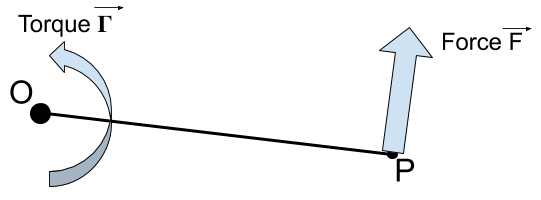Question #195

# What is the formula to calculate the force generated by a given torque?

Merged questions
AnswerThe formula to calculate the force created by a given torque is:

﻿﻿

With :

• ﻿﻿ is the force expressed in newtons [N]
• ﻿﻿ is the torque expressed in newtons-meters [N.m]
• ﻿﻿ is the radius expressed in meters [m], note that ﻿﻿ , the length of the OP segment.

This formula can also be expressed in the general case with the cross product:

﻿﻿

With :

• ﻿﻿ is the force vector expressed in newtons [N]
• ﻿﻿ is the torque vector expressed in newtons-meters [N.m]
• ﻿﻿ is the vector from rotation center ﻿﻿ to the point ﻿﻿ where the force ﻿﻿ is applied

3 events in history
Answer by Alphonsio 09/18/2020 at 05:48:42 PMThe formula to calculate the force created by a given torque is:

﻿﻿

With :

• ﻿﻿ is the force expressed in newtons [N]
• ﻿﻿ is the torque expressed in newtons-meters [N.m]
• ﻿﻿ is the radius expressed in meters [m], note that ﻿﻿ , the length of the OP segment.

This formula can also be expressed in the general case with the cross product:

﻿﻿

With :

• ﻿﻿ is the force vector expressed in newtons [N]
• ﻿﻿ is the torque vector expressed in newtons-meters [N.m]
• ﻿﻿ is the vector from rotation center ﻿﻿ to the point ﻿﻿ where the force ﻿﻿ is applied

Answer by Alphonsio 09/18/2020 at 05:48:25 PMThe formula to calculate the force created by a given torque is:

﻿﻿

With :

• ﻿﻿ is the force expressed in newtons [N]
• ﻿﻿ is the torque expressed in newtons-meters [N.m]
• ﻿﻿ is the radius expressed in meters [m], note that ﻿﻿ , the length of the OP segment.

This formula can also be expressed in the general case with the cross product:

﻿﻿

With :

• ﻿﻿ is the force vector expressed in newtons [N]
• ﻿﻿ is the torque vector expressed in newtons-meters [N.m]
• ﻿﻿ is the vector from rotation center ﻿﻿ to the point ﻿﻿ where the force ﻿﻿ is applied

Question by Alphonsio 09/18/2020 at 05:44:29 PM
What is the formula to calculate the force generated by a given torque?

Icons proudly provided by Friconix.